# Everything You Need to Know: VLOOKUP Exact Match

Oct 19, 2023Are you struggling to understand how to use VLOOKUP exact match?

You're not alone! Many people find this Microsoft Excel functionality a little hard to understand.

In this blog post, we'll explore what VLOOKUP exact match is all about and how it can help simplify tedious tasks like comparing two data sets.

Read on as we cover the following:

• What is VLOOKUP Function?

• How to use VLOOKUP Exact Match in Excel

• Final Thoughts on VLOOKUP Exact Match

• Frequently Asked Questions on VLOOKUP Exact Match## What is VLOOKUP Function?

VLOOKUP or the vertical lookup function in Excel helps people to quickly find and display data from a table without manually scanning or sorting through all the data. It's handy for tasks like finding a student's grade using their student ID or pulling up product details by entering a product code.

### How does it work?

VLOOKUP helps you search for a specific value in a large table or list of data. It looks for an exact or approximate match in the first column of that data. Once it finds a match, VLOOKUP retrieves information from other columns related to that match.

## VLOOKUP Function Syntax and Arguments

VLOOKUP syntax: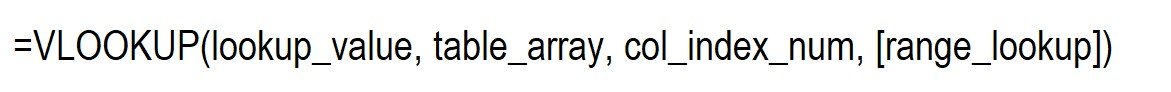VLOOKUP function arguments:

• Lookup value - the value to search. The lookup value can be (numeric, textual, or date value), cell reference with the lookup value, or the value retrieved by another Excel function.

• Table array - the data range to search the lookup value. The table array retrieves the matched value. The VLOOKUP function looks for information in the first column of a table. This column can have words, numbers, dates, and other things.

• Col index num - the column number where the lookup count starts. The col index num starts from the leftmost column of a table.

• Range lookup - the optional argument that determines whether to search for an approximate or exact match:

Range lookup parameter:

TRUE or the default value - is the approximate match. TRUE means it doesn't find an exact match, but the VLOOKUP formula looks for the largest value smaller than the lookup value or partial match.

It would be best if you sorted the lookup column in ascending order to use the TRUE parameter.

FALSE or the exact match - the VLOOKUP formula returns a FALSE value if a value is exactly equal to the lookup value.

If the VLOOKUP formula returns an "#N/A!" error value, the VLOOKUP formula doesn't find an exact value.

## How To Use VLOOKUP Exact Match in Excel

Here is a VLOOKUP example you can use to how to use VLOOKUP exact matches in Excel: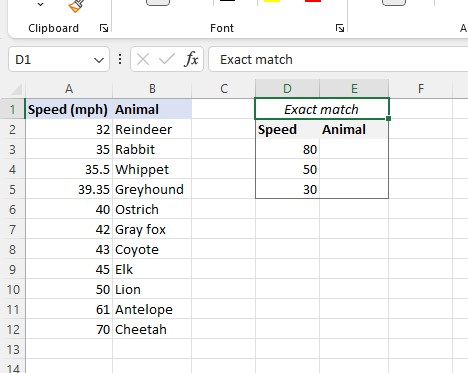1. Select a cell and type the VLOOKUP function.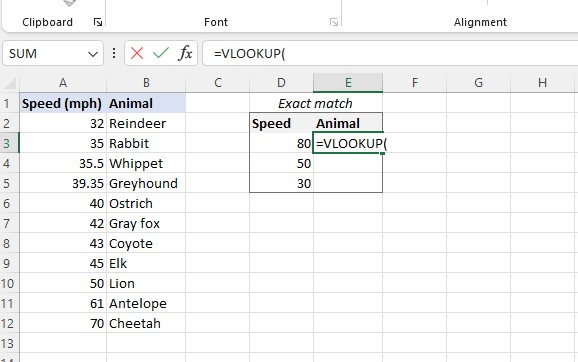1. Select the lookup value.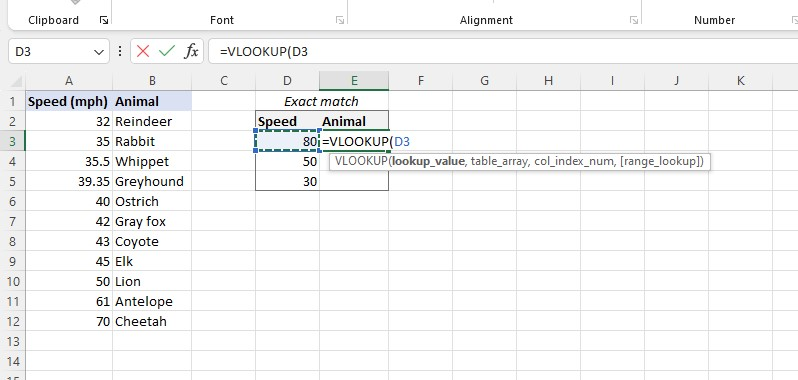1. Select your table array.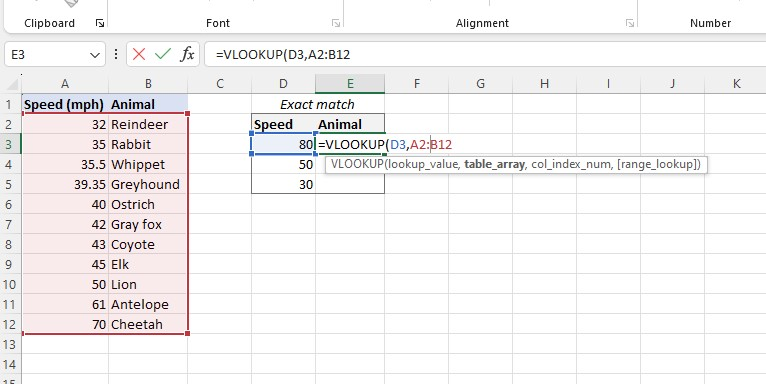1. Type "2" for the column index number to return a value from the second leftmost column.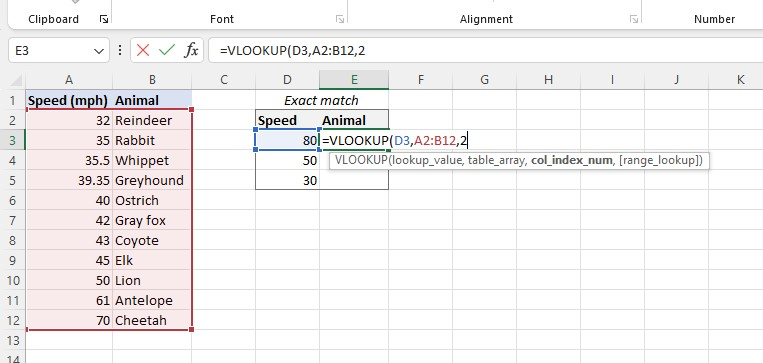1. Select "FALSE" for the range lookup to retrieve the exact match.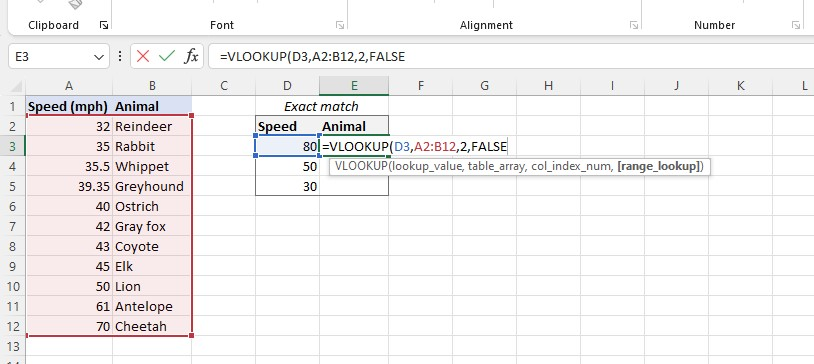1. After you've completed the VLOOKUP formula, press the enter key.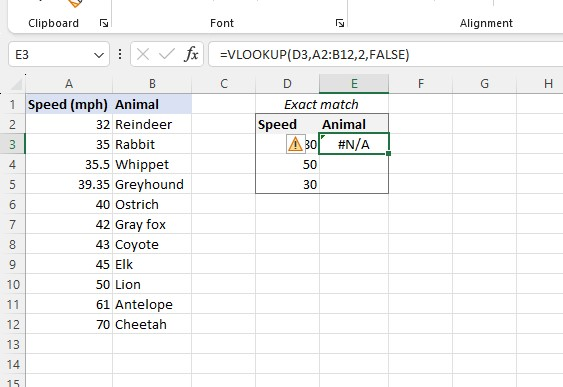The VLOOKUP formula returns an "#N/A!" error value because it can't find an exact match.By following the steps above, you can use the VLOOKUP formula to get the VLOOKUP value of the remaining cells.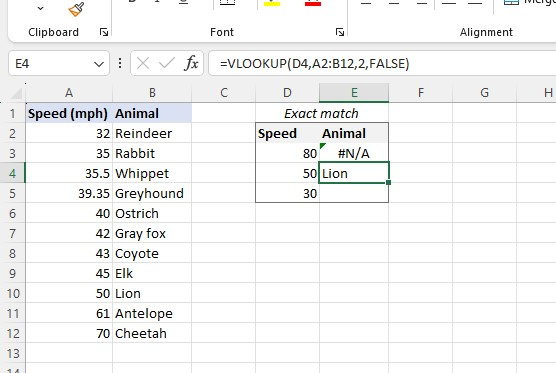## Final Thoughts on "VLOOKUP Exact Match"

Overall, the VLOOKUP exact match function is an incredibly powerful tool that has revolutionized how we search for information. This blog post demonstrates that it is fairly easy to use and drastically reduces the time spent looking for information in large data sets.

For the most straightforward Excel video tutorials, subscribe to Simple Sheets on YouTube!

## Frequently Asked Questions on "VLOOKUP Exact Match"

### What does "exact match" mean in VLOOKUP?

"Exact match" refers to finding an exact or identical match for the lookup value you provide. When using VLOOKUP with an exact match, the function will only return a result if it finds an exact match for the lookup value in the specified column.

### What if I have duplicate values in the lookup column when using VLOOKUP with an exact match?

If two of the same values are in a list, VLOOKUP will grab the first one it sees. It looks at the top of the column first.

### Can VLOOKUP be case-sensitive for an exact match?

No, by default, VLOOKUP is not case-sensitive for an exact match. It treats uppercase and lowercase letters as equivalent.## Related Articles:

Looking Up on Using VLOOKUPs?

The Only Guide You Need: VLOOKUP Multiple Columns

HLOOKUP In Excel: Secrets No One Has Told You

#### Want to Make Excel Work for You? Try out 5 Amazing Excel Templates & 5 Unique Lessons

We hate SPAM. We will never sell your information, for any reason.